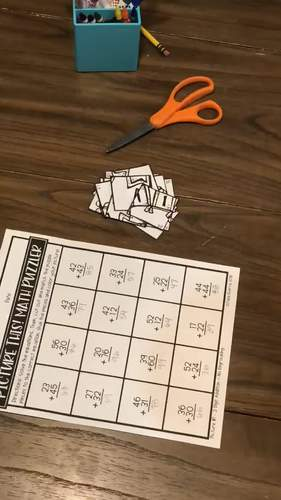# Picture This! Math Puzzles BUNDLESubject
Resource Type
File Type

Zip

(437 MB)
Standards
10 Products in this Bundle
10 products
• Bundle Description
• Standards

These differentiated math activities are the perfect addition and subtraction practice for your classroom! Each monthly resource includes 3 math puzzles with 8 differentiation options for each puzzle. I've also included an EDITABLE option for each puzzle! You can change the equations to fit the needs of your students. This makes differentiating for your students a breeze! All students can work on the same puzzle at varying levels.

Each puzzle includes the following differentiation options PLUS a completely editable option for each puzzle:

• Mixed Addition and Subtraction to 18
• 2 Digit Addition no regrouping
• 2 Digit Addition with regrouping
• 2 Digit Subtraction no regrouping
• 2 Digit Subtraction with regrouping
• 3 Digit Subtraction

*You must have MS Powerpoint for the editable puzzle option.

Check out these individual Monthly Math Puzzles:

Back to School Math Puzzles / Addition and Subtraction Puzzles

October Math Puzzles / October Math Activities

January Math Puzzles / Addition and Subtraction Puzzles

February Math Puzzles / Addition and Subtraction Puzzles

Earn Credits Towards Future Purchases:

If you like this product, please leave feedback at my TPT store. Each time you leave feedback, you earn credits that can be used towards your future TPT purchase! Hooray!

Let's Connect!

To keep up with the latest on new products, sales, and flash freebies, connect with me on:

…………………………………………………………………………………………………

Copyright © 2018 Jana Guerra at We Heart Teaching. All rights reserved by author. Permission to copy for single classroom use only. Electronic distribution limited to single classroom use only. Not for public display.

Fluently add and subtract within 20 using mental strategies. By end of Grade 2, know from memory all sums of two one-digit numbers.
Add and subtract within 20, demonstrating fluency for addition and subtraction within 10. Use strategies such as counting on; making ten (e.g., 8 + 6 = 8 + 2 + 4 = 10 + 4 = 14); decomposing a number leading to a ten (e.g., 13 - 4 = 13 - 3 - 1 = 10 - 1 = 9); using the relationship between addition and subtraction (e.g., knowing that 8 + 4 = 12, one knows 12 - 8 = 4); and creating equivalent but easier or known sums (e.g., adding 6 + 7 by creating the known equivalent 6 + 6 + 1 = 12 + 1 = 13).
Fluently add and subtract multi-digit whole numbers using the standard algorithm.
Fluently add and subtract within 1000 using strategies and algorithms based on place value, properties of operations, and/or the relationship between addition and subtraction.
Fluently add and subtract within 100 using strategies based on place value, properties of operations, and/or the relationship between addition and subtraction.
Total Pages
N/A
Included
Teaching Duration
N/A
Report this Resource to TpT
Reported resources will be reviewed by our team. Report this resource to let us know if this resource violates TpT’s content guidelines.